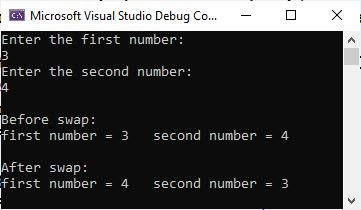# C# program to swap two numbers

This post was published to provide the information and program in C# that are used to swap two numbers. I have created two C# programs, one without user input and the other with user input.

Let me clear up one thing: I am not going to use any third or temporary variable to swap two numbers.

## Simple C# program to swap two numbers

Now let me create a simple C# program that swaps two numbers without using the third variable. Also, I am not allowing users to input any data through this program.

```int numOne = 10;
int numTwo = 20;

Console.WriteLine("Before swap:");
Console.WriteLine("'numOne' = " + numOne + "   'numTwo' = " + numTwo);

numOne = numOne + numTwo;
numTwo = numOne - numTwo;
numOne = numOne - numTwo;

Console.WriteLine("\nAfter swap:");
Console.WriteLine("'numOne' = " + numOne + "   'numTwo' = " + numTwo);```

The output produced by this C# example should exactly be

```Before swap:
'numOne' = 10   'numTwo' = 20

After swap:
'numOne' = 20   'numTwo' = 10```

## C# program to swap two numbers entered by user

This C# program is created in a way to receive two numbers as input from the user at run-time of the program. Then those two numbers will be swapped and printed back on the output console.

```int a, b;

Console.WriteLine("Enter the first number: ");
Console.WriteLine("Enter the second number: ");

Console.WriteLine("\nBefore swap:");
Console.WriteLine("first number = " + a + "   second number = " + b);

a = a + b;
b = a - b;
a = a - b;

Console.WriteLine("\nAfter swap:");
Console.WriteLine("first number = " + a + "   second number = " + b);```

The output produced by this C# program with user inputs 3 and 4 is shown in the snapshot given below.C# Online Test

« Previous Program Next Program »